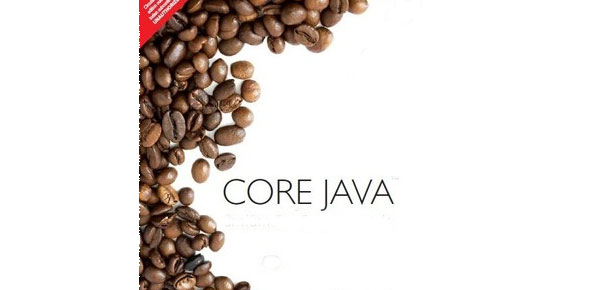# Essentials Of Core Java

35 Questions | Attempts: 99SettingsQuiz based on must know concepts of Core Java covering almost all essential aspects of programming language. Make sure you come out with flying colors :)Passing: 70%Time: 30 Minutes

• 1.
Is it possible to have abstract static method for a class?
• A.

Yes

• B.

No

• 2.
The return type of constructor must always be void. True or False?
• A.

True

• B.

False

• 3.
______ command is used to invoke Java compiler
• A.

Javac

• B.

Javacomp

• C.

Java

• D.

Jc

• 4.
Which of the following methods are from Thread class:
• A.

Sleep

• B.

Wait

• C.

Yield

• D.

Join

• 5.
CoreJava java = new CoreJava(){ public void learn(){ System.out.println("YavaJavaYavaJava"); } };Above code is an example of:
• A.

Anonymous inner class

• B.

Serialization

• C.

• D.

Collection

• 6.
The generic Type information does not exist at runtime. All generic code is strictly for compiler. This process of removing type information out of the class bytcode is called "Type Erasure". True or False?
• A.

True

• B.

False

• 7.
Which of the following are examples of sorted collection?
• A.

ArrayList

• B.

TreeSet

• C.

TreeMap

• D.

TreeList

• 8.
ArrayList
• A.

Implements RandomAccess (marker) interface

• B.

Is an ordered but unsorted collection

• C.

Is faster than vector

• D.

Does not allow duplicate elements

• 9.
Which of the following is/are true statements regarding equals method?
• A.

If X equals Y and Y equals Z then X equals Z

• B.

X.equals(null) will return false

• C.

Null.equals(x) will return false

• D.

Null.equals(x) will give NullPointerException

• 10.
If you want to save the state of your object, you can implement ________ interface
• A.

Runnable

• B.

Callable

• C.

Serializable

• D.

Clonable

• 11.
What would be the output:String website = "www.YavaYavaJavaJava.com"; System.out.println(s.substring(4,20));
• A.

YavaYavaJavaJava

• B.

.YavaYavaJavaJava.

• C.

AvaYavaJavaJava

• D.

Www.YavaYavaJavaJava.com

• 12.
How many String objects would be created in following code:String s1 = "YavaYava"; String s2 = "JavaJava"; String s3 = ".com"; s1 = "www." + s1 + s2 + s3; String s4 = new String("www.YavaYavaJavaJava.com");
• A.

5

• B.

4

• C.

6

• D.

1

• 13.
Which of the following is/are true statement(s)?
• A.

StringBuffer is slower than StringBuilder

• B.

StringBuffer is synchronized while StringBuilder is not

• C.

Strings are immutable objects

• D.

Always use StringBuffer instead of StringBuilder

• 14.
assert keyword was introduced in Java version ____
• A.

1.4

• B.

1.3

• C.

1.6

• D.

1.7

• 15.
Which of the following are checked exceptions?
• A.

NullPointerException

• B.

ArrayIndexOutOfBoundsException

• C.

IllegalStateException

• D.

FileNotFoundException

• 16.
Is below code valid?public void getValue(){ try{ System.out.println("Hi"); }catch(OutOfMemoryError e){ System.out.println("Error occured"); }finally{ System.out.println("Finally always executes"); } }
• A.

Yes

• B.

No

• 17.
Which of the below for loops are valid?
• A.

For ( ; ; ;){}

• B.

For (int i = 0; i ++){}

• C.

For (int i = 0; i < 10; i--){ }

• D.

For (i < 10)

• 18.
What would be the output of below code:String string = "Hi"; switch(string.length()){ case 1: System.out.print("One "); case 2: System.out.print("Two "); case 3: System.out.print("Three "); default: System.out.print("Default "); }
• A.

Two

• B.

One Two Three Default

• C.

One Two Three

• D.

Two Three Default

• 19.
_____________ operator can be used to check if object is of particular type
• A.

Instanceof

• B.

InstanceOf()

• C.

Isinstanceof

• D.

IsInstance

• 20.
Convert below code using ternary operators:String x = ""; if(i > 10){ x = "Hello"; }else { x = "World" }
• A.

String x = (i > 10) ? "Hello" : "World";

• B.

String x = (i > 10) : "Hello" ? "World";

• C.

String x = (i > 10) @ "Hi" : "Hello";

• D.

String x = (i > 10) = "World" ? "Hello";

• 21.
___________ method is a mechanism to run some code just before object is deleted by garbage collector.
• A.

Finalize

• B.

ToString

• C.

Finally

• D.

Equals

• 22.
Which of the following is/are correct statement(s)
• A.

Instance variables live on heap

• B.

Local variables live on stack

• C.

Local variable and instance variables live on heap

• D.

Objects live on heap

• 23.
Methods can be overloaded or overriden while constructors can
• A.

• B.

Be overriden

• C.

• D.

Not be overriden

• 24.
Good Object Oriented design should
• A.

Have loose coupling but high cohesion

• B.

Have loose coupling and low cohesion

• C.

Have tight coupling and high cohesion

• D.

Have tight coupling but low cohesion

• 25.
____________ Allows code defined in one class to be reused in other classes
• A.

Inheritance

• B.

Abstraction

• C.

Poly Morphism

• D.

Encapsulation

## Related TopicsBack to top
×

Wait!
Here's an interesting quiz for you.